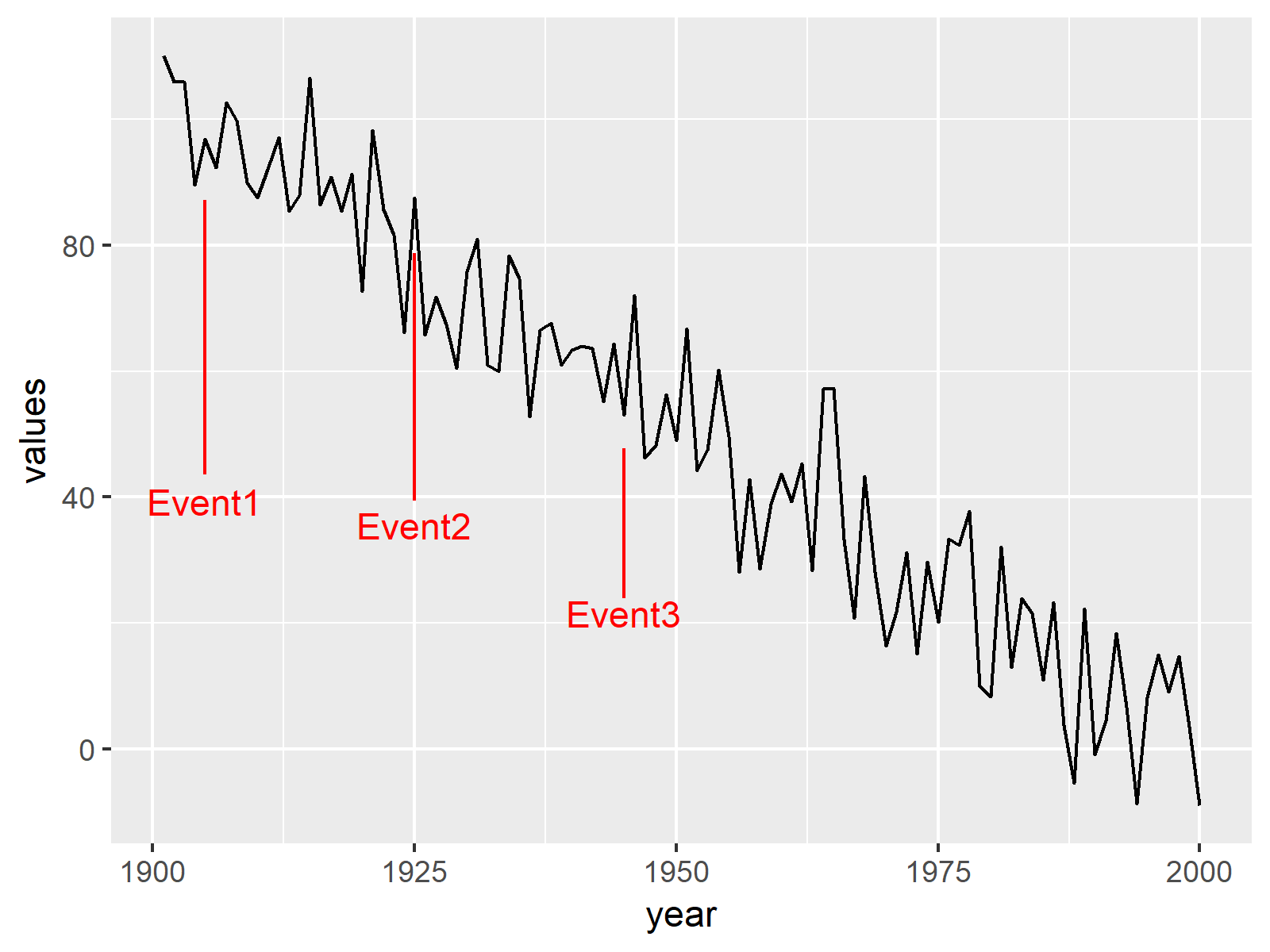# Draw Time Series Plot with Events Using ggplot2 Package in R (Example)

This post shows how to create a time series graph containing specific events in the R programming language.

So without further ado, let’s dive right into the example!

## Example Data

First, we have to create a data frame containing some example values for a time series:

```set.seed(98213674)                                     # Example data for time series
data_values <- data.frame(year = 1901:2000,
values = 100:1 + rnorm(100, 0, 10))
#   year    values
# 1 1901 110.18375
# 2 1902 105.93849
# 3 1903 106.02063
# 4 1904  89.64547
# 5 1905  96.89598
# 6 1906  92.29945```

Have a look at the previous output of the RStudio console. It visualizes that our example data consists of two columns. One variable contains the time line of our time series in years and the other variable contains the corresponding values.

We also have to create a second data frame consisting of the events and the corresponding years. Note that the following R code is also creating two variables defining the starting and ending points of segment lines that we will add later to the plot.

```data_events <- data.frame(year = c(1905, 1925, 1945),  # Example data for events
name = c("Event1", "Event2", "Event3"))
data_events\$yend <- data_values\$values[data_values\$year %in% data_events\$year] * 0.9
data_events\$ystart <- data_events\$yend * 0.5
data_events                                            # Print events
#   year   name     yend   ystart
# 1 1905 Event1 87.20639 43.60319
# 2 1925 Event2 78.75749 39.37874
# 3 1945 Event3 47.71781 23.85891```

## Example: Creating Time Series Plot with Events

This Example shows how to use the ggplot2 package to create a time series plot with events. We first need to install and load the ggplot2 package, if we want to use the functions that are included in the package:

```install.packages("ggplot2")                            # Install ggplot2 package

Now, we can use the ggplot, geom_line, geom_segment, and geom_text functions to create our time series graphic. Note that we are specifying NULL within the ggplot function, since we are creating the ggplot2 plot based on two data frames.

```ggp <- ggplot(NULL) +                                  # Create ggplot2 time series graph
geom_line(data = data_values, aes(x = year, y = values)) +
geom_segment(data = data_events, aes(x = year, y = ystart, xend = year, yend = yend), color = "red") +
geom_text(data = data_events, aes(x = year, y = ystart * 0.9, label = name), color = "red")
ggp                                                    # Draw plot```The output of the previous R programming syntax is shown in Figure 1 – As expected, we created a time series plot with certain events.

## Video, Further Resources & Summary

In case you want to learn more about the ggplot2 package and data visualization in R, please have a look at the following video tutorial, where I give a detailed introduction with different examples:

Please accept YouTube cookies to play this video. By accepting you will be accessing content from YouTube, a service provided by an external third party.If you accept this notice, your choice will be saved and the page will refresh.

In addition, you could have a look at the related tutorials on my website.

You learned in this post how to plot a time series with events in the R programming language. In case you have additional questions, let me know in the comments section.

Subscribe to the Statistics Globe Newsletter

•Fran
October 20, 2022 7:43 pm

Thank you.

It does not work if you use autoplot and define data frame as a time series

•November 14, 2022 12:38 pm